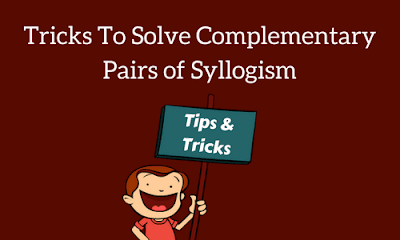# Tricks To Solve Complementary Pairs of SyllogismStatement:
All P are Q
• Possibility conclusion of “ALL”:
• Some Q is not P is a possibility.
• All Q is P is a possibility.

Statement:
Some P is Q

Possibility case:
• Some P is not Q is a possibility.
• All p is Q is a possibility
• All Q is P is a possibility
• Some Q is not P is a possibility.

Statement:
No P is Q
• There is no possibility in No statement. If the conclusion comes with NO, then there is no possibility occur.

Statement:
Some P is not Q
Conclusion:
• Some Q is p is a possibility.
• All Q is P is a possibility.
• No Q is P is a possibility.
• Some Q is not P is a possibility.

## Exceptional Case:

1) When there is no relation found between the statements, there is always a possibility true.
2) Some not/ some not reserved + anything = No conclusion
For Example
Statement:
Some P is Q
Some Q is R

As you know,
Some + Some = No conclusion
But if in conclusion,
Some P being R is a possibility.
Some P is R.
In the above statement
The First conclusion is right because in it there is a word written possibility, while the second Conclusion is wrong because it is the absolute conclusion.

## What is a Complimentary pair?

A complimentary pair is a type in which both conclusions are not true at a time and are not false at the same time.
So, from the both conclusions one have to be true while the other has to be wrong.
When the answer given like either 1 follows or 2 follows, it is a case of complimentary syllogism.

### Types of a complementary pair:

There are three different types of complementary pairs.
• Some + some not
• Some + no
• All + some not

Let’s look at complementary conclusions.
• All + some not
• All P are Q
• Some P are Not Q
• Some + no
• Some P is Q
• No P is Q
• Some + Some not
• Some P is Q
• Some P are not Q

#### Example

1) Statement:
All pen are Pencils
Some Pencils are Book

Conclusion:
A: All Pencils are Pen
B: No Pen is book
C: Some book is pen

Options
A) Only Conclusion A Follows
B) Only Conclusion B follows
C) Either Conclusion A or B follows
D) Either Conclusion B or C follows
E) None of these follows
Solution: D
2) Statement:
Some Pen is Pencils
All Pencils are Book

Conclusion:
A: All book are pencils
B: Some Pen is not Book
C: All Pen is book
Options
A) Only Conclusion A Follows
B) Only Conclusion B follows
C) Either Conclusion A or B follows
D) Either Conclusion B or C follows
E) None of these follows
Solution: D

3) Statement:
All Pen are pencils
Some Pen is Book
No Book is Eraser

Conclusions:
A: Some pen being Eraser is a possibility
B: Some Pen is Eraser

Options
A) Only Conclusion A Follows
B) Only Conclusion B follows
C) Either Conclusion A or B follows
D) Either Conclusion B or C follows
E) None of these follows
Solution: C